# MCQs on Electric Circuits

##### Page 54 of 63. Go to page 1 2 3 4 5 6 7 8 9 10 11 12 13 14 15 16 17 18 19 20 21 22 23 24 25 26 27 28 29 30 31 32 33 34 35 36 37 38 39 40 41 42 43 44 45 46 47 48 49 50 51 52 53 54 55 56 57 58 59 60 61 62 63
01․ The rms value of the resultant current in a wire which carries a DC current of 10A and a sinusoidal current of peak value 20A is
14.14A.
22.4A.
17.3A .
30A.

Rms value of sinusoidal current = 20/√2 = 10√2 So, RMS value of resultant current is given by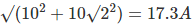02․ 2) Two sinusoidal emfs are given as e1 = Asin(wt+45) and e2 = Bsin(wt-30). The phase difference between the two quantities in degrees is
75.
60.
15.
105.

The phase difference is given by 45-(-30) = 75 degrees.

03․ Which of the following statements pertains to resistors only?
Act as energy storage devices.
Dissipate desirable amount of power.
They oppose sudden change in voltages.
None of these.

Resistors are linear bilateral passive elements that resist the flow of current and thereby dissipate power in the circuit.

04․ The capacitive reactance of an electric circuit is 50 Ohms. The frequency of supply becomes doubled. What is the new capacitive reactance?
200 Ohms.
100 Ohms.
50 Ohms.
25 Ohms.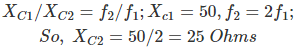05․ The inductive reactance of an electric circuit is 200 Ohms. The frequency of supply becomes doubled. What is the new capacitive reactance?
50 Ohms.
100 Ohms.
200 Ohms.
400 Ohms.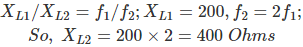06․ In which of the circuits power factor can be zero?
capacitive.
inductive.
both ‘a’ and ‘b’
resistive.

Power factor will be zero when Cosθ = 0 and this is possible only in capacitive and inductive circuits.

07․ The active and reactive power in an AC circuit are 10W and 20VAR respectively. What is the power factor?
0.866.
0.5.
0.577.
1.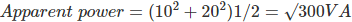Power factor = active power/apparent power = 10/√300 = 0.577.

08․ In the above question find the angle between voltage and current?
30.
54.74.
60.
90.

Power factor = cosθ = 0.577; θ = 54.74 degrees.

09․ The frequency of EMF generated by a generator depends upon its
both speed and number of poles.
speed.
number of poles.
nature of supply.

As frequency of generated EMF = Number of poles × Speed/120

10․ A voltage of v = 100 sin (wt + π/3) is applied to pure resistor of 30 Ohm. What is the RMS value of current is
4.71.
5.77.
1.92.
2.36.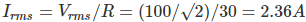<<<5253545556>>>